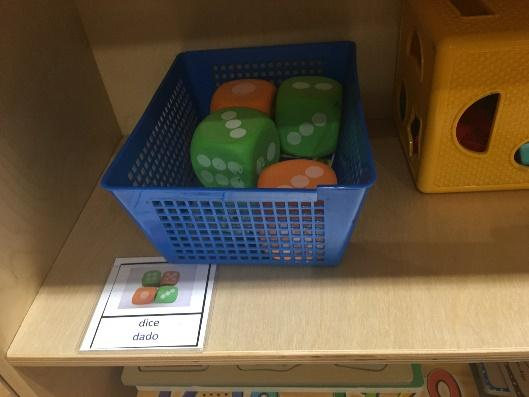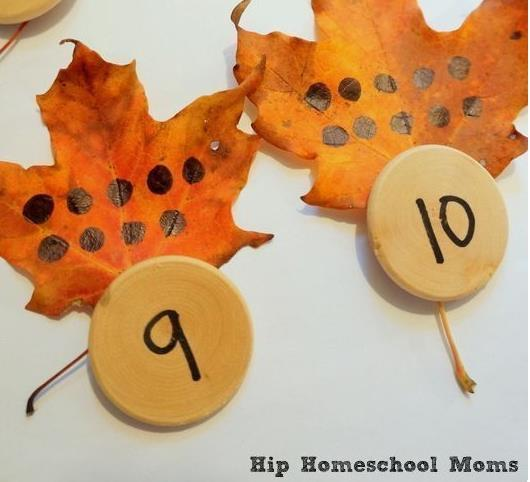## 0012: How to SLAY ECERS3-math Items Part 1

Important Math Facts:

1. Early Math skills are predictive of later math AND reading achievement.
1. First-hand experience with hands-on materials is CRUCIAL to later math learning. Infants and toddlers must be given ample opportunities to explore objects and their environment.
1. Children learn math through problem-solving, reasoning, communicating, making connections, and representing (Copley, 2000; Guiest, 2009) in Creative Curriculum Vol. 6, sixth ed.
1. Language development and the ability to understand words is a critical foundational piece of math learning.
• Exhaustive List of Skills:
• Counting
• Ordering
• Comparing
• Classifying
• Identifying
• Predicting
• Estimating
• Adding
• Subtracting
• Dividing
• Matching
• Determining Parts /Whole
• Sequencing
• Measuring
• Predicting how many
• Predicting how long
• Predicting how far
• Predicting how high
• Describing characteristics of shapes
• Sorting shapes
• Matching shapes
• Measuring
• Comparing
• Matching
• Classifying
• Sorting
• By Color
• By Shape
• By Size
• By more than one attribute

What MATH CONCEPTS do you see?

Counting, 1:1 correspondence; Matching numerals with # of objects; Sorting and Classifying, Identifying shapes; identifying numerals; measuring; patterns

What MATH SKILLS do you see?

Counting, 1:1 correspondence; Matching numerals with # of objects; Sorting and Classifying,  Identifying numerals; measuring; patterning, seriation, measuring weights, subitizing, adding, subtracting

Number Concepts and Operations:

• What does “3” mean?
• Where do children see numbers in their environments? (besides on number games and toys)
• What can you ask a child,  to find out if he understands the meaning of a number that you happen to see while playing?

Connecting numerals with their quantities

Being able to identify numerals in the environment

Identifying numerals within play and activities

Connecting numbers to their counted objects

Understanding and using place value and base ten –

Counting

• Knowing the sequence of the numbers, 1,2,3,4….etc.
• 1:1 correspondence
• Ordinal counting: first, second, third, fourth, in sequence
• Counting on from a quantity
• Counting back
• Counting by groups (2,4,6,8,10…)

Quantity

• Cardinality – understanding the idea of “quantity” – when I count 1,2,3,4, the quantity is 4.
• the written symbol, or numeral, should be presented with the correct grouping, to help children associate the amount with the numeral (Copley, 200; Payne and Huinker, 1993).
• How many
• More / Less / the same (equal)

Subitizing

• Being able to look at a small group of objects and identify the quantity without counting (such as when looking at dice or dominoes)• More / less
• How many
• Parts and wholes

Separating – subtracting; “taking away” – “How many are left?”

Combining – adding – “How many in all?”

• Using their fingers has been shown to be an effective way to learn adding and subtracting
• Using physical objects that are directly related to the problems being solved are most effective (Clements & Sarama, 2009) in Creative Curriculum Vol. 6, sixth ed.

Ages 5-6, children begin to be able to solve addition and subtraction word problems

Adding and subtracting small numerals on a white board – ages 5-7

Sorting and Classifying

Comparing

What Opportunities for developing MATH CONCEPTS or MATH SKILLS do you see here?

Spatial Relationships and Shapes

Spatial awareness – understanding how objects are oriented in relation to one another

Positional Words describe spatial relationships:

• Location words: In, out, on, off, under, above, over, next to, by, upside-down
• Directional words describe “which way” – up, down, forward, backward
• Following directions related to positions
• Follow directions related to proximity
• Using positional words indicating location, direction, and distance
• Making simple maps, sketches, models to depict and locate objects

Shapes:

• 2-dimenional shapes: square, triangle, circle, rectangle, diamond, etc.
• 3-dimensional shapes: cube, cylinder, sphere, rectangular prism
• Shapes can be maneuvered to appear in different ways, rotated into different positions
• Shapes can be compared
• Children need to draw, manipulate, describe, sort, and represent shapes in a variety of ways
• Putting shapes in a shape sorter
• Drawing a car made out of shapes
• Working on a shape puzzle
• Combining two shapes to make another shape (2 squares make a rectangle)

Shapes awareness; comparing shapes; describing shapes; positional awareness; matching

Measurements and Comparisons

Sizes

Seriation: ordering from smallest to largest

Putting things in order

• By size, length, weight

Measuring things using non-standard units of measure, including paper clips, cubes, links, hands, feet, string

Understanding the purpose of standard measuring tools

Can measure things using standard units of measure such as ruler, measuring tape, or scale

Uses measurement words such as heavier, lighter, longer, shorter, weighs

Ordering, sequencing, measuring, counting, matching numerals to quantities

Weighing, comparing, predicting, experimenting, counting, describing, evaluating what happened

Time – understanding the basic sequence of the daily events

Uses some time words and tools – calendar, clock

Counting; measuring; sorting; patterning;

Using ordinal numbers – first, next, last, second, third

Reading graphs – comparing picture graphs

Using graphs to represent data

Patterns

Patterns; sequencing; ordering

Shapes awareness; spatial awareness; counting; parts of the whole; patterning; sizes

Shapes awareness; spatial awareness; counting; parts of the whole; sequencing

Counting, 1:1 correspondence; Matching numerals with # of objects; Sorting and Classifying, Identifying numerals; measuring; patterning, seriation, measuring weights, subitizing, adding, subtracting

Counting, ordering, matching numerals to quantities, subitizing, ordering, 1:1 correspondence

1:1 correspondence,  matching numerals to quantities; concepts of a little, some, more, most, empty, fullMatching numerals to quantities

Parts of the whole; counting, adding, quantities

Shapes awareness; positions

Shapes awareness; positions; parts of whole

Shapes awareness; positions; parts of whole

1:1 correspondence; counting

Videos:

Math related Videos: The following short videos will give you an even greater understanding of how very young children begin to develop their understanding of number and math concepts and skills.  This is important foundational experience for them.

https://www.zerotothree.org/resources/250-everyday-fun-with-shapes-let-s-talk-about-math-video

https://www.zerotothree.org/resources/254-everyday-fun-with-measurement-let-s-talk-about-math-video

https://www.zerotothree.org/resources/2224-let-s-talk-about-math-making-math-language-part-of-everyday-routines

https://www.zerotothree.org/resources/series/let-s-talk-about-stem-video-series

https://www.zerotothree.org/resources/252-everyday-fun-with-counting-let-s-talk-about-math-video

https://www.zerotothree.org/resources/253-everyday-fun-with-addition-and-subtraction-let-s-talk-about-math-video

https://www.zerotothree.org/resources/series/let-s-talk-about-math-early-math-video-series

Links:

https://www.zerotothree.org/early-learning/early-math-and-science

https://www.zerotothree.org/resources/2225-got-math-20-tips-for-making-your-trip-to-the-grocery-store-count

https://www.zerotothree.org/resources/299-help-your-child-develop-early-math-skills

https://www.zerotothree.org/resources/382-a-good-fit